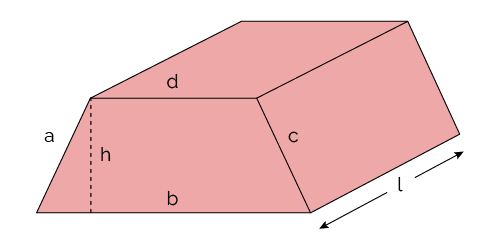# Lateral Area Trapezoidal Prism Calculator

Created by Wei Bin Loo
Reviewed by Rijk de Wet
Last updated: Jun 05, 2023

With this lateral area trapezoidal prism calculator, we aim to help you to calculate the lateral area of a trapezoidal prism. You can check out our surface area calculator and quadrilateral calculator to understand more about this topic.

We have written this article to help you understand what the lateral area of a trapezoidal prism is and how to calculate the lateral area trapezoidal prism. We will also demonstrate some examples to help you understand the the concept.

## How to find the lateral area of a trapezoidal prism?

The lateral area is the sum of the areas of all the sides of an 3D object besides the base and the top. Check out our rectangular prism calculator for more information.

To understand the calculation of the lateral area of a trapezoidal prism, let's take the following trapezoidal prism as an example:

• Side a: 4 m
• Side b: 7 m
• Side c: 4 m
• Side d: 3 m
• Length (ℓ): 10 m
• Height (h): 7 m

You can calculate the lateral area of a trapezoidal prism in four steps:

1. Determine the length (ℓ)

In this example, the length of the trapezoidal prism is 10 m.

2. Determine the height (h)

For our example, the height of the trapezoidal prism is 7 m.

3. Determine the lengths of sides a, b, c, and d

You can see sides a, b, c and d in the diagram. For this trapezoidal prism, these lengths are 4 m, 7 m, 4 m, and 3 m respectively.

4. Compute the volume of the trapezoidal prism

The last step is to compute the lateral area using the following formula:

lateral area = ℓ × (a+b+c+d)

Hence, the lateral area of this trapezoidal prism is 10 m × (4 m + 7 m + 4 m + 3 m) = 180 m².

## How to calculate the surface area of a trapezoidal prism?

After understanding how to find the lateral area of a trapezoidal prism, let's talk about surface area. The surface area is the sum of the areas of all the sides of an 3D object, incising its base and top.

For the surface area calculation, we will use the same trapezoidal prism as the last example.

You can calculate the surface area of a trapezoidal prism in four steps:

1. Determine the length (ℓ)

The same as before, the length of the trapezoidal prism is 10 m.

2. Determine the height (h)

The height of the trapezoidal prism is 7 m.

3. Determine the lengths of sides a, b, c, and d

Following the diagram, you can see the side a, side b, side c, and side d of the trapezoidal prism are 4 m, 7 m, 4 m, and 3 m respectively.

4. Calculate the surface area of the trapezoidal prism

The last step is to compute the surface area using the following formula:

surface area = h×(b+d) + ℓ×(a+b+c+d)

Hence, the surface area of this trapezoidal prism is 7 m × (7 m + 3 m) + 10 m × (4 m + 7 m + 4 m + 3 m) = 250 m².

## FAQ

### How do I calculate the lateral area of a trapezoidal prism?

You can calculate the lateral area of a trapezoidal prism in four steps:

1. Determine the length (ℓ)
2. Determine the height (h)
3. Calculate side a, side b, side c and side d
4. Apply the surface area formula: lateral area = ℓ × (a + b + c + d)

### How do I calculate the surface area of a trapezoidal prism?

You can calculate the surface area of a trapezoidal prism in four steps:

1. Determine the length (ℓ)
2. Determine the height (h)
3. Calculate side a, side b, side c and side d
4. Apply the surface area formula: surface area = h × (b + d) + ℓ × (a + b + c + d)

### Can lateral area be negative?

No, lateral area cannot be negative. This is because zero and negative lateral area simply doesn't exist. An object cannot have zero or negative lateral area.

### What is the lateral area of a trapezoidal prism with all 1 m sides?

The lateral area of that trapezoidal prism will be 4 m². You can calculate it by using this formula: lateral area = length * (side a + side b + side c + side d).

Wei Bin Loo
SIde a
m
Side b
m
Side c
m
Side d
m
Length (ℓ)
m
Height (h)
m
Lateral area
Surface areaPeople also viewed…

### Circle skirt

Circle skirt calculator makes sewing circle skirts a breeze.

### Complex number to polar form

Complex number to polar form calculator helps you rewrite a complex number from rectangular to polar form.

### Free fall

Our free fall calculator can find the velocity of a falling object and the height it drops from.

### Round to the nearest tenth

Round to the nearest tenth calculator is your new best friend to help to round decimal numbers to the nearest tenth.